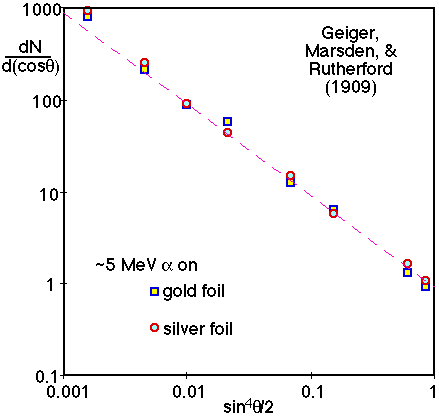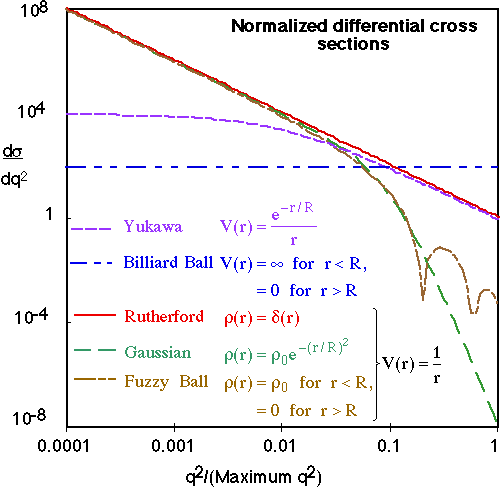Quantum Scattering

Born approximation: incident particle is a plane wave and scattered amplitude is a spherical wave.

ytot = yinc + yscatt = ei(pz-Et) + f(q) ei(pr-Et)/r

In general, the differential cross-section can be written as

ds/dW = |f(q)|2

where f(q) is essentially the Fourier transform of the scattering potential

f(q) = -(m/2p)V(r) eiq·r d3r

If the scattering is spherically symmetric

f(q2) = -(m/2p)V(r) r sin(qr) dr

If V(r)~1/r, then simple dimensional counting requires f(q2)~1/q2.

Rutherford scattering can be analysed either classically or quantum mechanically.

(ds/dW)R = 4m2(Z1Za)2/q4

In general, if there is no range or size scale in a scattering problem, then dimensional analysis requires

ds/dq2 µ 1/q4 Þ ds/dW µ 1/sin4 (q/2)

Which is very different from the the differential cross section expected from billiard ball scattering (ds/dW µ constant).For electromagnetic scattering of incident point particles, we can consider scattering from an extended target as point-point scattering integrated over the charge distribution of the target:

ds/dW = (ds/dW)R |F(q)|2

The Form Factor is the Fourier transform of the target's charge distribution:

F(q2) =r(r) eiq·r d3r

and (ds/dW)R is the reference cross section for poin-point scattering (e.g. For electron scattering this is usually Mott scattering, but Rutherford scattering can be used in the low energy limit.)

Comparison of differential cross sections for scattering a spinless point particle from either a Rutherford point particle or particles with a size R=10/qmax.Electron-proton scattering

F&H Figure 6.13: the proton is not a point electric charge, but has a size ~1fm.

F&H Figure 6.5: atomic nuclei have a size and a reasonable well defined edge.

F&H Figure 6.19: protons do not have a well defined edge, but inside the proton there seem to be point charge constituents.